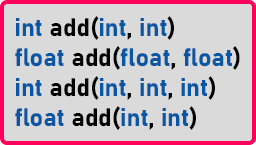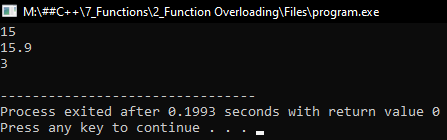In C++, we can write more than one function with the same name but with a different argument or parameter list, and when we do so, it is called function overloading. Let us understand this with an example.
void main(){
int a = 10, b = 2, c;
}

This is our main function. Inside this function, we have declared 3 variables. Next, we are storing the result of the ‘add()’ function in the ‘c’ variable. The following is the add function.
return x + y;
}

Here we haven’t declared any variable, simply return ‘x + y’. When we call the ‘add’ function inside the main function, then ‘a’ will be copied in ‘x’ and ‘b’ will be copied in ‘y’ and it will add these two numbers and the result will store in ‘c’. Now we want to write one more function here,
int add(int x, int y, int z){
return x + y + z;
}

We have changed the main function as follows.
void main(){
int a = 10, b = 2, c, d;
d = add (a, b, c);
}

Here we have created another function of the same name that is ‘add’ but it is taking 3 parameters. Inside the main function, we have called ‘add(x,y,z)’ and stored the result in the ‘d’ variable. So, we can have two functions with the same name but with different parameters

So when we call ‘add(a, b)’ it will be calling add(int x, int y) and when we call ‘add(a, b, c)’ it will be add(int x, int y, int z). C++ compiler can differentiate between these two functions, and this is the concept of function overloading in C++.

The benefit here is that we don’t have to think of new names every time. As both the functions are for adding integers, so we don’t have to give different names. It is easy for writing the programs and you don’t have to remember too many function names. That is the benefit we are getting now. Let us declare one more add function which returns the sum of two float numbers.

return x + y;
}

This function will return the float type value. Now we have two add functions that are taking the same number of parameters. Is it possible in C++? Yes, two functions can have the same name and the same number of parameters but the data type of the parameters should be different. They cannot be the same.

So int add (int x, int y) and float add (float x, float y) are two different functions. In C++ two functions are said to be different if they have the same name but different parameters list.

##### How the parameters list can be different?

Either the data type of the parameter or the number of parameters. For better understanding, please have a look at the below image.Above are the signatures of the different ‘add’ functions. Now let us check which is valid or which is invalid.

• int add(int, int) is valid, it is taking 2 ‘int’ type parameters and return ‘int’ value.
• float add(float, float) is valid as it is taking 2 ‘float’ parameters and return the ‘float’ value. It is taking the same number of parameters but different data types as compared to the first one.
• int add(int, int, int) is valid as it is taking 3 ‘int’ parameters and return ‘int’ value. It is taking a different number of parameters but has the same data types as compared to the first one.
• float add(int, int) is invalid, it is the same as the first function which is both the function taking the same number of parameters and the same type of parameters. So, this is invalid. It doesn’t matter what type of data a function is returning. If two functions have the same number of parameters and of the same types then this is invalid.

```#include<iostream>
using namespace std;
int Sum(int a, int b)
{
return a + b;
}

float Sum(float a, float b)
{
return a + b;
}

int Sum(int a, int b, int c)
{
return a + b + c;
}

int main()
{
cout << Sum(10, 5) << endl;
cout << Sum(12.5f, 3.4f) << endl;
cout << Sum(10, 20, 3) << endl;
return 0;
}
```
###### Output:##### What is a signature/prototype?

The header of a function is called a signature or prototype.
Example: int fun(int x,float y);

##### Two functions with the same name. Are they overloaded?

Yes, they are overloaded functions if their parameters are different.

No.

##### Two functions with the same name and parameters, but different return types. Are they overloaded?

Example:
int fun(int x, int y)
float fun(int x, int y)

1.